Function Repository Resource:

# RandomMetalPseudoSubgenre

Generate a new metal subgenre in the spirit of the many metal microgenres

Contributed by: Christopher Stover
 ResourceFunction["RandomMetalPseudoSubgenre"][] generates a String consisting of one random (probably-fictitious) metal subgenre. ResourceFunction["RandomMetalPseudoSubgenre"][n] generates a List of n subgenres. ResourceFunction["RandomMetalPseudoSubgenre"][UpTo[n]] is the same as ResourceFunction["RandomMetalPseudoSubgenre"][n]. ResourceFunction["RandomMetalPseudoSubgenre"][{n1,n2,…}] generates an n1×n2×⋯ array of subgenres.

## Details

In the above, the arguments n and ni must be integers.
ResourceFunction["RandomMetalPseudoSubgenre"] automatically threads over lists of integers.
ResourceFunction["RandomMetalPseudoSubgenre"] uses RandomInteger and RandomSample under the hood to create as much randomness as possible, but duplicates may still exist (see "Possible Issues" below).

## Examples

### Basic Examples (1)

Generate a random (probably-fictitious) metal subgenre:

 In:=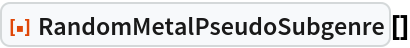Out=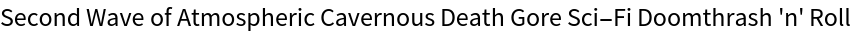### Scope (3)

Here are five random (probably-fictitious) metal subgenres:

 In:=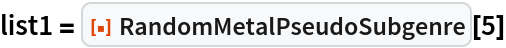Out=It may be easier to read as a Column:

 In:=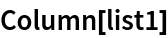Out=Using UpTo provides a different way to get five random (probably-fictitious) metal subgenres:

 In:=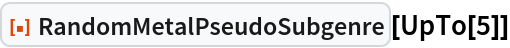Out=Here is an array of random (probably-fictitious) metal subgenres:

 In:=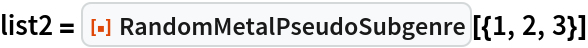Out=The nesting is easier to visualize using Grid with the option:

 In:=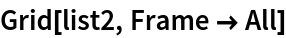Out=### Possible Issues (2)

RandomMetalPseudoSubgenre[n] returns unevaluated when n is any data type not mentioned in "Usage":

 In:=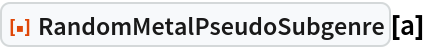Out=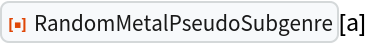In:=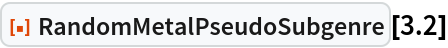Out=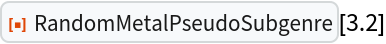In:=Out=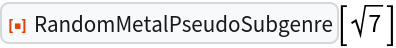In:=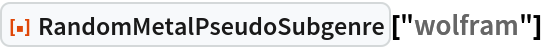Out=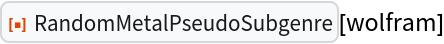RandomMetalPseudoSubgenre uses RandomInteger and RandomSample under the hood to create as much randomness as possible. For small samples, this is generally enough to avoid duplicates:

 In:=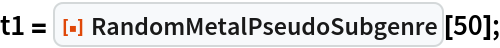In:=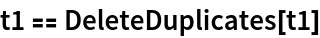Out=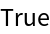Even so, duplicates may still exist in larger examples:

 In:=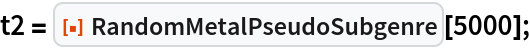In:=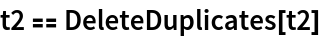Out=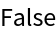In:=Out=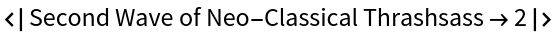### Neat Examples (6)

While all of the examples are neat, some stand out more than others. For example, sometimes, you get really long subgenre names:

 In:=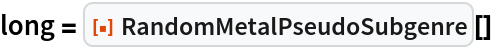Out=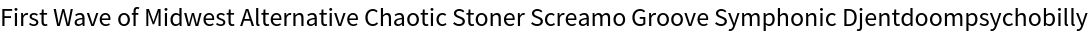In:=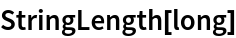Out=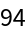And sometimes, the subgenre names are really short:

 In:=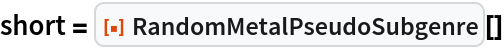Out=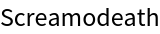In:=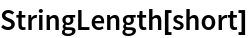Out=On average, however, the lengths of the subgenre names tend to be more average. Experimental evidence suggests that the Mean and Median of the subgenre name lengths are both around 50:

 In:=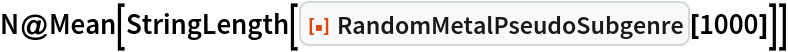Out=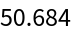In:=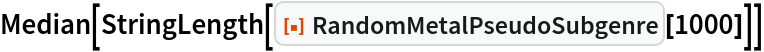Out=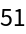To make metal seem more academic, you can test the Mean/Median hypothesis by using StringLength on longer and longer lists of subgenres. This yields a pair of datasets (where x is the length of the subgenre list and y is the Mean/Median of the corresponding list's string lengths) which seem to support the hypothesis.

Here is a plot of the corresponding Mean data:

 In:=Out=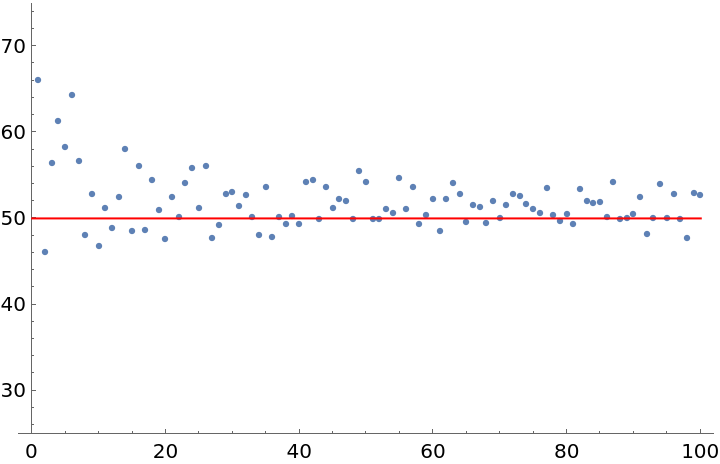And here is one for the Median data:

 In:=Out=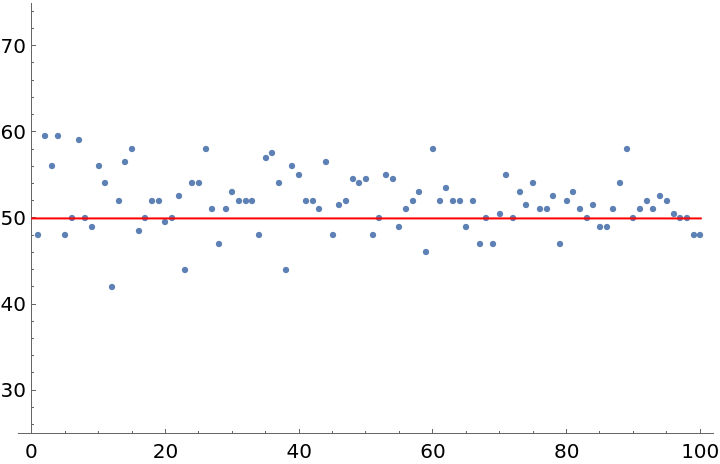The trend can be summarized by taking the Mean of the means and the Median of the medians. Again, the results are very close to 50:

 In:=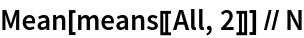Out=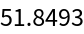In:=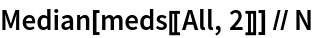Out=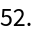therealcstover

## Version History

• 1.0.0 – 14 March 2022

## Author Notes

There are several other notable metal genre prefixes that could have gone into the lists used herein; however, some prefixes deemed either "trivial" or "obscene" were omitted.
There is a great deal of musical knowledge built in to the Wolfram Language. As a starting point, one can check the entities listed under the "Music" subsection at Guide: Cultural Data.